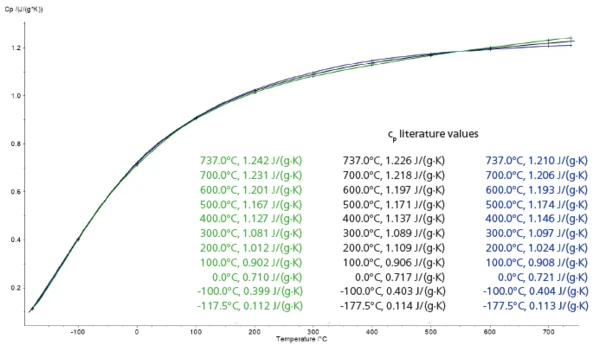# Precise Determination of the Specific Heat Capacity in a Wide Temperature Range by Means of DSC

#### Introduction

The Specific Heat Capacity (cp)Heat capacity is a material-specific physical quantity, determined by the amount of heat supplied to specimen, divided by the resulting temperature increase. The specific heat capacity is related to a unit mass of the specimen.specific heat capacity of a material is the quantity of heat necessary to raise the temperature of the unit mass of material by 1 K at a constant temperature . For the determination of the heat capacity of a material in accordance with DIN ISO 11357-4 by DSC, three measurements are required: a blank run with two empty crucibles; a calibration measurement using, for example, a sapphire disk; and the sample measurement. Accurate Specific Heat Capacity (cp)Heat capacity is a material-specific physical quantity, determined by the amount of heat supplied to specimen, divided by the resulting temperature increase. The specific heat capacity is related to a unit mass of the specimen.specific heat capacity results are only achieved if all three runs are carried out under exactly the same conditions. This is only possible if the curves delivered by the DSC device are characterized by high reproducibility.

#### Measurement Conditions

The Specific Heat Capacity (cp)Heat capacity is a material-specific physical quantity, determined by the amount of heat supplied to specimen, divided by the resulting temperature increase. The specific heat capacity is related to a unit mass of the specimen.specific heat capacity of two different sapphire disks was determined between -177.5°C and 737°C, i.e., over a temperature range of almost 915°C. Both sapphire sample were measured under the same conditions. The Specific Heat Capacity (cp)Heat capacity is a material-specific physical quantity, determined by the amount of heat supplied to specimen, divided by the resulting temperature increase. The specific heat capacity is related to a unit mass of the specimen.specific heat capacity of each sapphire was determined by using the other one as a standard. Such a wide temperature in the low-temperature application can only be achieved with the DSC 300 Caliris® equipped with the socalled H-Module. Table 1 depicts the conditions of the measurements.

Table 1: Measurement condition1) Comparison of the specific heat data of sapphire with literature values 

Table 2: Comparison of the measurement results with literature 

#### Test Results

Figure 1 shows the specific heat curves for both sapphire samples (sample 1, green; sample 2, blue) compared with the curve taken from literature (black) . It not only demonstrates that the repeatability of the measurements is very good, but also shows their accuracy. For the temperature range spanning from -177.5°C to 737°C, the specific heat values determined by means of the

DSC 300 Caliris® are in very good agreement with literature data (maximum error: 1.75%; see table 2). This excellent result was only achievable thanks to the good reproducibility of the DSC measurements.

#### Literature

1. 
2. 
NBS Certificate, Standard Reference Material 720, Synthetic Sapphire